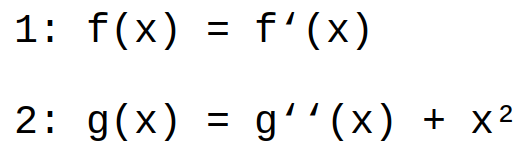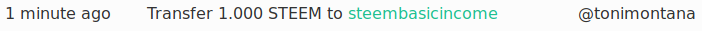# Math contest #8 Results and Solution

in #puzzlelast year

## Solution:

The problem of the last contest was:1. solution:
`f(x) = df(x)/dx |×dx ÷f(x)`
`dx = 1/f(x) × df(x) |integrate`
`x + C = ln(f(x)) |e^()`
`f(x) = e^(x+C)`
2. solution:
Assumption: `g(x) = x²+h(x)`
`g''(x) = h''(x) + (x²)'' = h''(x) + 2`
`h(x) = h''(x) + 2`
`h(x) = i(x)+2`
`i(x) = i''(x)`
Assumption: `i(x) = e^(kx)`
`e^(kx) = k²*e^(kx)`
`k² = 1``k = ±1`
`i(x) = A*e^x + B*e^-x`
`g(x) = x² + 2 + A*e^x + B*e^-x`

↓↑↓↑↓↑↓↑↓↑↓↑↓↑↓↑↓↑↓↑↓↑↓↑↓↑↓↑↓↑↓↑↓↑↓↑↓↑↓↑↓↑↓↑↓↑↓↑↓

### How your chance of winning is calculated:

• Every participant gets 1 point for entering the contest.
• Every solution entered gets 1 additional point.
• Multiple examples of a general solution count as one solution
• If there is only one general solution, those who found its pattern(by mentioning a general formula or showing more then 3 examples) will get an additional point.
• Your winning chance is `"your points"/"total points"`

↓↑↓↑↓↑↓↑↓↑↓↑↓↑↓↑↓↑↓↑↓↑↓↑↓↑↓↑↓↑↓↑↓↑↓↑↓↑↓↑↓↑↓↑↓↑↓↑↓
All participants did great and got the maximum score, but I list the score anyway.

### List of participants with their scores and chance of winning, sorted by time of entry:

Namesolutions foundscorechance of winning
@tonimontanag(x)=c1*e^x+c2*e^(-x)+x^2+23100%

Judging by the participants it seems like differential equation is not a thing for this contest. So I'll go back to normal equation.

↓↑↓↑↓↑↓↑↓↑↓↑↓↑↓↑↓↑↓↑↓↑↓↑↓↑↓↑↓↑↓↑↓↑↓↑↓↑↓↑↓↑↓↑↓↑↓↑↓
Congratulations @tonimontana, you won 1 SBI:↓↑↓↑↓↑↓↑↓↑↓↑↓↑↓↑↓↑↓↑↓↑↓↑↓↑↓↑↓↑↓↑↓↑↓↑↓↑↓↑↓↑↓↑↓↑↓↑↓

### The next contest starts tomorrow. Don't miss it!

Sort:

Differential equations are scary for normal Steemians...

Yes I think you are right. I also do not like solving differential equations very much.